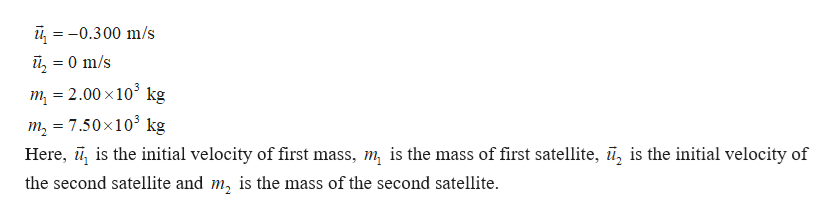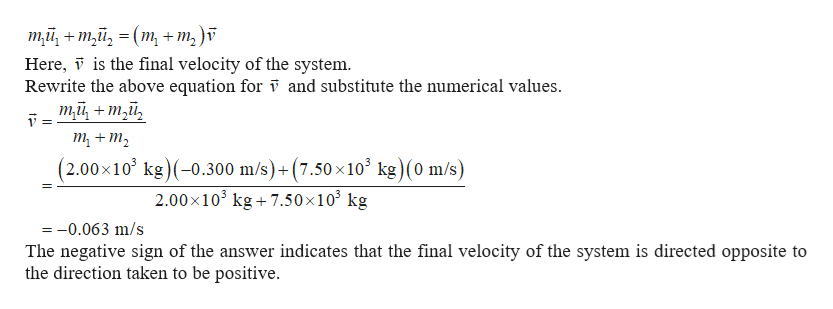Two manned satellites approaching one another at a relative speed of 0.300 m/s intend to dock. The first has a mass of 2.00 ✕ 103 kg, and the second a mass of 7.50 ✕ 103 kg. If the two satellites collide elastically rather than dock, what is their final relative velocity? Adopt the reference frame in which the second satellite is initially at rest and assume that the positive direction is directed from the second satellite towards the first satellite.  m/s

Question

Two manned satellites approaching one another at a relative speed of 0.300 m/s intend to dock. The first has a mass of 2.00 ✕ 103 kg, and the second a mass of 7.50 ✕ 103 kg. If the two satellites collide elastically rather than dock, what is their final relative velocity? Adopt the reference frame in which the second satellite is initially at rest and assume that the positive direction is directed from the second satellite towards the first satellite.
m/s

Step 1

Since the reference frame in which the second satellite is at rest is chosen, the initial velocity of the second satellite will be zero. Since the second satellite is at rest, the relative speed between the two satellites will be equal to the magnitude of velocity of the first satellite.  Since positive direction is assumed to be from the second satellite to the first satellite, the initial velocity of the first satellite will be negative. Thus, the given quantities can be written ashelp_outlineImage Transcriptionclose-0.300 m/s îl 0 m/s = m = 2.00 x103 kg m 7.50x103 kg Here, , is the initial velocity of first mass, m is the mass of first satellite, ū, is the initial velocity of = the second satellite and m, is the mass of the second satellite fullscreen
Step 2

Since the satellites are collided elastically, the momentum of the system will b...help_outlineImage Transcriptioncloseтй, + т,й, 3 (т, + m,)f Here, T is the final velocity of the system Rewrite the above equation for 7 and substitute the numerical values. mй, + т,й, т, + т, (2.00x10 kg) (-0.300 m/s)+(7.50 x103 kg)(0 m/s) 2.00x103kg7.50x103 kg =0.063 m/s The negative sign of the answer indicates that the final velocity of the system is directed opposite to the direction taken to be positive. fullscreen

Want to see the full answer?

See Solution

Want to see this answer and more?

Our solutions are written by experts, many with advanced degrees, and available 24/7

See Solution
Tagged in

Kinematics